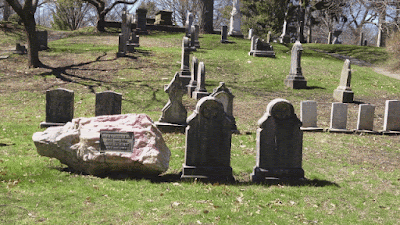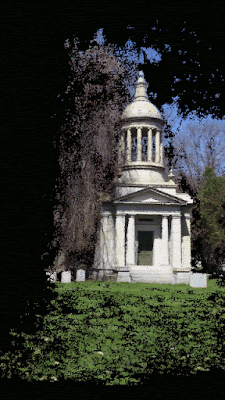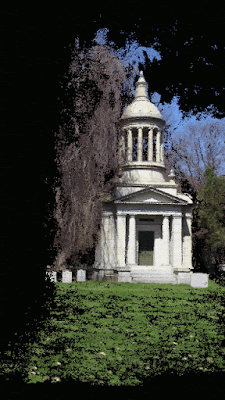## Friday, May 20, 2016

### Depth Map Automatic Generator 5 (DMAG5) - Downsampling factor

As you probably know by now, Depth Map Automatic Generator 5 (DMAG5), the leading automatic depth map generator from ugosoft3d, has a new parameter which downsamples the input images in order for the whole process of generating depth maps to be (much) faster. Let's see the impact of this "downsampling factor" in the quality of the depth/disparity maps produced by DMAG5.

Let's generate depth maps with DMAG5 using a downsampling ratio/factor of 1 (no downsampling), 2, and 4.

This takes 07:40 (7 minutes 40 seconds) of cpu time using debug version of DMAG5 on a linux box.

This takes 00:53 (53 seconds) of cpu time using debug version of DMAG5 on a linux box. This is a speedup of 8.7 when compared to cpu time without downsampling.

This takes 00:07 (7 seconds) of cpu time using debug version of DMAG5 on a linux box. This is a speedup of 65.7 when compared to cpu time without downsampling.

Of course, as the downsampling ratio/factor increases, the depth map quality decreases a bit but isn't a good speedup worth a little bit of depth map quality degradation? At the very least, it's a great to see the effects of the parameters on the generated depth/occlusion maps.Animated gif created by wigglemaker using the depth map obtained with a downsampling factor equal to 2.

## Thursday, May 19, 2016

### 3D Photos - Gilbert pink granite stone

This shows how to generate a depth map and an animated 3d gif from two unaligned pictures.Input: two pictures taken from a mono camera, in this case, extracted from a video taken by an iphone4. The image dimensions are 1920x1080.

Step 1: Rectify the images using Epipolar Rectification 9b (ER9b)

This is the input to ER9b:
IMG_0069_04.jpg
IMG_0069_03.jpg
output_04.jpg
output_03.jpg
10000

step 2: Generate the depth/disparity map using Depth Map Automatic Generator 5 (DMAG5)

This is the input to DMAG5:
output_04.jpg
output_03.jpg
-17
65
disp1.jpg
disp2.jpg
occ1.jpg
occ2.jpg
32
0.9
30.0
10.0
4
0
9
9.0
25.5
2

The min and max disparities were obtained from the output of ER9b. In order to speed up the disparity map generation process, a downsampling factor of 2 was used (instead of 1). The left disparity should be such that white means foreground and black means background. If it appears inverted, the easiest thing to do is to go back to step 1, switch the order of the input images, and recompute the depth maps.

Step 3: Generate the animated gif using wigglemaker

Before attempting to generate the animated gif, it's a good idea to reduce the size of the reference image (left image) and corresponding disparity map.

If you want to generate tweeners (in-between frames) to make a lenticular or another kind of animated gif, Frame Sequence Generator 6 (FSG6) can be used. Now, for FSG6, the right depth map must be such that foreground is white and background is black which is not what DMAG5 outputs. In other words, the right depth map that DMAG5 outputs must be inverted prior to using FSG6.

## Wednesday, May 18, 2016

### Multi View Stereo - Magazine rack at Target

Here's an example of a 3d dense reconstruction obtained with Structure from Motion 10 (SfM10) and Multi View Stereo 10 (MVS10).

The parameters used for MVS10 were:
- Number of trials used in rectification = 10000
- Minimum average separation angle (camera pair selection) = 0.5
- Radius used to smooth the cost = 32
- Alpha = 0.9
- Truncation value for color cost = 30.0
- Truncation value for gradient cost = 10.0
- Epsilon = 4
- Disparity tolerance used to detect occlusions = 0
- Downsampling factor = 4
- Sampling step = 1
- Minimum separation angle = 0.5
- Minimum number of image points per 3D point = 3
- Maximum reprojection error = 2.0
- Radius for the animated gif frames = 1

### Multi View Stereo - Gardening aisle at Target

Here's an example of a 3d dense reconstruction obtained with Structure from Motion 10 (SfM10) and Multi View Stereo 10 (MVS10).

The parameters used for MVS10 were:
- Number of trials used in rectification = 10000
- Minimum average separation angle (camera pair selection) = 0.5
- Radius used to smooth the cost = 32
- Alpha = 0.9
- Truncation value for color cost = 30.0
- Truncation value for gradient cost = 10.0
- Epsilon = 4
- Disparity tolerance used to detect occlusions = 0
- Downsampling factor = 4
- Sampling step = 1
- Minimum separation angle = 0.5
- Minimum number of image points per 3D point = 4
- Maximum reprojection error = 2.0
- Radius for the animated gif frames = 1

### Multi View Stereo - Davenport burial plot

Here's an example of a 3d dense reconstruction obtained with Structure from Motion 10 (SfM10) and Multi View Stereo 10 (MVS10).

The parameters used for MVS10 were:
- Number of trials used in rectification = 10000
- Minimum average separation angle (camera pair selection) = 0.5
- Radius used to smooth the cost = 32
- Alpha = 0.9
- Truncation value for color cost = 30.0
- Truncation value for gradient cost = 10.0
- Epsilon = 4
- Disparity tolerance used to detect occlusions = 0
- Downsampling factor = 4
- Sampling step = 1
- Minimum separation angle = 0.5
- Minimum number of image points per 3D point = 3
- Maximum reprojection error = 2.0
- Radius for the animated gif frames = 1

### Multi View Stereo - Whitney mausoleum

Here's an example of a 3d dense reconstruction obtained with Structure from Motion 10 (SfM10) and Multi View Stereo 10 (MVS10).

The parameters used for MVS10 were:
- Number of trials used in rectification = 10000
- Minimum average separation angle (camera pair selection) = 0.5
- Radius used to smooth the cost = 32
- Alpha = 0.9
- Truncation value for color cost = 30.0
- Truncation value for gradient cost = 10.0
- Epsilon = 4
- Disparity tolerance used to detect occlusions = 0
- Downsampling factor = 4
- Sampling step = 1
- Minimum separation angle = 0.5
- Minimum number of image points per 3D point = 4
- Maximum reprojection error = 2.0
- Radius for the animated gif frames = 1

### Multi View Stereo - Winged cherub

Here's an example of a 3d dense reconstruction obtained with Structure from Motion 10 (SfM10) and Multi View Stereo 10 (MVS10).

The parameters used for MVS10 were:
- Number of trials used in rectification = 10000
- Minimum average separation angle (camera pair selection) = 0.5
- Radius used to smooth the cost = 32
- Alpha = 0.9
- Truncation value for color cost = 30.0
- Truncation value for gradient cost = 10.0
- Epsilon = 4
- Disparity tolerance used to detect occlusions = 0
- Downsampling factor = 4
- Sampling step = 1
- Minimum separation angle = 0.5
- Minimum number of image points per 3D point = 4
- Maximum reprojection error = 2.0
- Radius for the animated gif frames = 1

## Friday, May 6, 2016

### Multi View stereo - Gilbert pink granite stone

Let's see the effect of the max reprojection error (low-confidence image points) on the 3D reconstruction produced by Multi View Stereo 10 (MVS10). If the reprojection error of an image point is too large, it is assumed the image point is not reliable and if the number of image points goes below the minimum number of image points, then it is the 3D point itself that's considered unreliable.

Let's start with max reprojection error (low-confidence image points) = 2. Incidentally, we are using min separation angle (low-confidence 3D points) = 0.5 and min image point number (low-confidence 3D points) = 3. Those are fixed.

MVS10 outputs:
Number of 3D points = 1,300,510
Number of 3D points removed because behind ref camera = 23,295
Number of 3D points removed because separation angle too low = 270,093
Number of 3D points removed because too few image points = 635,181
Number of 3D points = 371,941

Let's continue with max reprojection error (low-confidence image points) = 4 and see what happens to the 3D reconstruction.

MVS10 outputs:
Number of 3D points = 1,220,135
Number of 3D points removed because behind ref camera = 22,258
Number of 3D points removed because separation angle too low = 206,778
Number of 3D points removed because too few image points = 412,677
Number of 3D points = 578,422

As expected, when the max reprojection error (low-confidence image points) is raised, the final number of 3D points in the 3D reconstruction increases. Are those extra 3D points erroneous? They don't appear to be, at least when the 3D reconstructions are viewed in animated gif form.

### Multi View Stereo - Whittemore mausoleum

Let's see the effect of the min separation angle (low-confidence 3D points) on the 3D reconstruction produced by Multi View Stereo 10 (MVS10). If the separation angle of a 3D point is too low, it is kind of assumed that its position is not too accurate and it should probably not be in the dense 3D reconstruction.

Let's start with min separation angle (low-confidence 3D points) = 0.5. Incidentally, we are using min image point number (low-confidence 3D points) = 3 and max reprojection error (low-confidence image points) = 2. Those are fixed.

MVS10 outputs:
Number of 3D points = 1,042,740
Number of 3D points removed because behind ref camera = 23,384
Number of 3D points removed because separation angle too low = 42,701
Number of 3D points removed because too few image points = 663,332
Number of 3D points = 313,323

Let's move on with min separation angle (low-confidence 3D points) = 1.5 and see what happens to the 3D reconstruction.

MVS10 outputs:
Number of 3D points = 1,042,740
Number of 3D points removed because behind ref camera = 23,384
Number of 3D points removed because separation angle too low = 261,161
Number of 3D points removed because too few image points = 446,460
Number of 3D points = 311,755

Interestingly enough, when the min separation angle is increased, the number of 3D image points that are removed because the separation angle is too low is increased but the number of 3D points that are removed because there are too few image points (fewer than 3) is decreased by about the same amount and the number of 3D points that remain is about the same. In any case, the two dense 3D reconstructions seem to be quite similar, at least, when viewed as animated gifs.

### Multi View Stereo - Mausoleum

Let's see if the downsampling factor that is used to compute disparity maps in Multi View Stereo 10 (MVS10) has much of an impact on the quality of the dense 3D reconstruction. It's important to know because computing disparity maps is quite time consuming, especially in MVS10 which computes two depth maps per image pair (to detect low-confidence disparities and therefore matches).Set of eight 1080x1920 images/views for which we want to build a dense 3D reconstruction with MVS10.Animated gif showing the dense 3D reconstruction produced by MVS10 using downsampling factor = 4 (and sampling step = 2).

A downsampling factor of 4 means that the original images are downsampled (shrunk) by a factor of two twice. This dense 3D reconstruction has 351,520 3D points.Animated gif showing the dense 3D reconstruction produced by MVS10 using downsampling factor = 2 (and sampling step = 2).

A downsampling factor of 2 means that the original images are downsampled (shrunk) by a factor of two once. This dense reconstruction has 384,808.

Looking at the two animated gifs, there doesn't seem to be much of a difference between the two reconstructions. The conclusion is that downsampling images in order to compute disparity maps faster is a-ok (as long as you don't overdo it and downsample too much).

## Thursday, May 5, 2016

### Multi View Stereo - Celtic cross

The input to Structure from Motion 10 (SfM10) is a set of six 1080x1920 images extracted from a video taken with a iphone 4s. Once the Structure from Motion part is done (computation of the camera poses and of the sparse 3D reconstruction of the scene), the "nvm" file (output of SfM10) is fed to Multi View Stereo 10 (MVS10) which constructs the dense 3D reconstruction of the scene. The output of MVS10 is an animated gif and a "ply" file (point cloud file format) of the dense reconstruction.